### Home > PC > Chapter 4 > Lesson 4.3.1 > Problem4-124

4-124.
1. Find all values of θ that satisfy the equations below. Assume 0 ≤ θ ≤ 2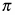. Use exact values. Homework Help ✎1. sin θ =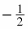2. cos θ =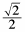3. tan θ =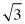4. csc θ =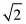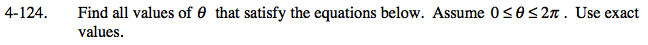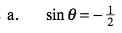Draw a unit circle. In which quadrants is sine negative?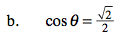1. What special angle is this?
2. In which quadrants is cosine positive?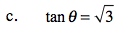$\tan(\theta)=\frac{\sin(\theta)}{\cos(\theta)}$

$\tan(\theta)=\frac{\sqrt{3}}{1}=\frac{\sqrt{3}/2}{1/2}$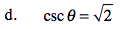$\frac{1}{\sin(\theta)}=\frac{\sqrt{2}}{1}$